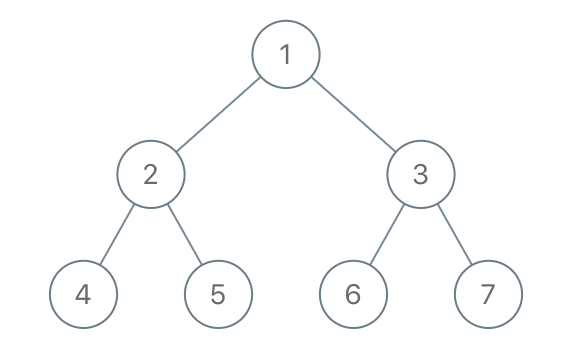# 1110. Delete Nodes And Return ⭐ Forest

Medium
Given the `root` of a binary tree, each node in the tree has a distinct value.
After deleting all nodes with a value in `to_delete`, we are left with a forest (a disjoint union of trees).
Return the roots of the trees in the remaining forest. You may return the result in any order.
Example 1:Input: root = [1,2,3,4,5,6,7], to_delete = [3,5]
Output: [[1,2,null,4],,]
Example 2:
Input: root = [1,2,4,null,3], to_delete = 
Output: [[1,2,4]]
Constraints:
• The number of nodes in the given tree is at most `1000`.
• Each node has a distinct value between `1` and `1000`.
• `to_delete.length <= 1000`
• `to_delete` contains distinct values between `1` and `1000`.

### 解題

/**
* Definition for a binary tree node.
* type TreeNode struct {
* Val int
* Left *TreeNode
* Right *TreeNode
* }
*/
func delNodes(root *TreeNode, to_delete []int) []*TreeNode {
m := make(map[int]bool)
for _, n := range to_delete {
m[n] = true
}
ans := make([]*TreeNode, 0)
var dfs func(*TreeNode, bool) *TreeNode
dfs = func(r *TreeNode, isRoot bool) *TreeNode {
if r == nil { return nil }
if isRoot && !m[r.Val] {
ans = append(ans, r)
}
r.Left = dfs(r.Left, m[r.Val])
r.Right = dfs(r.Right, m[r.Val])
if m[r.Val] { return nil }
return r
}
dfs(root, true)
return ans
}